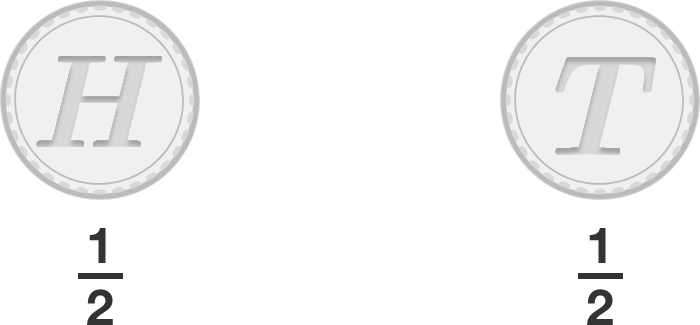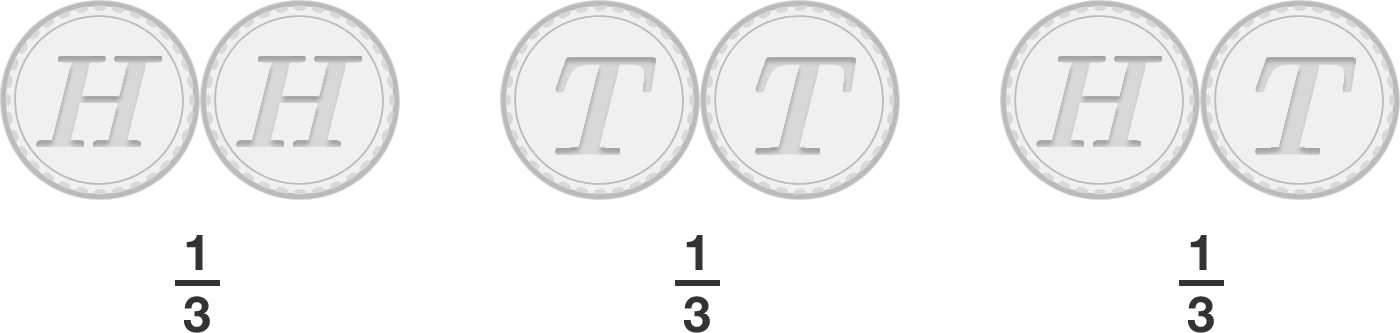# Number of outcomes is all that matters

Probability Level 1

True or False?

If I toss a fair coin once, then the only possible outcomes are heads or tails with equal probabilities. So the probability of obtaining a head from a single toss is $\frac12$.If I toss a fair coin twice, then the only possible outcomes are "2 heads," "2 tails" or "1 head and 1 tail," again with equal probabilities. So the probability of obtaining "1 head and 1 tail" from two tosses is $\frac13$.×

Problem Loading...

Note Loading...

Set Loading...